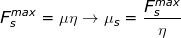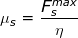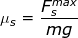# Static Friction Formula

Static Friction Formula

Static friction is a force that keeps an object at rest. It must be overcome to start moving the object. Once an object is in motion, it experiences kinetic friction. If a small amount of force is applied to an object, the static friction has an equal magnitude in the opposite direction. If the force is increased, at some point the value of the maximum static friction will be reached, and the object will move. The coefficient of static friction is assigned the Greek letter "mu" (μ), with a subscript "s". The maximum force of static friction is μs times the normal force on an object.

force of static friction ≤ (coefficient of static friction)(normal force) maximum force of static friction = (coefficient of static friction)(normal force)

Fs ≤ μs η , and Fsmax = μs η

Fs = force of static friction

μs = coefficient of static friction

η = normal force (Greek letter "eta")

≤ means "less than or equal to"

Fsmax = maximum force of static friction

Static Friction Formula Questions:

1) A 5500 N force is applied to a sled full of firewood in a snow-covered forest. The skis of the sled have a coefficient of static friction μs = 0.75 with the snow. If the fully-loaded sled has a mass of 700 kg, what is the maximum force of static friction, and is the force applied enough to overcome it?

Answer: On a flat surface, the normal force on an object is η = mg. Using this, the maximum force of static friction can be found:

Fsmax = μs η

Fsmax = μs mg

Fsmax = (0.75)(700 kg)(9.8 m/(s2))

Fsmax = 5145 kg∙m/s2

Fsmax = 5145 N

The maximum force of static friction is 5145 N, and therefore the applied force of 5500 N is enough to overcome it, and start moving the sled.

2) A person building a brick-making machine wants to measure the coefficient of static friction between brick and wood. To do this, she places a 2.00 kg brick on a flat piece of wood, and gradually applies more and more force until the brick moves. She finds that the brick moves when exactly 11.8 N of force is applied. What is the coefficient of static friction?

Answer: The force that was applied was exactly the right amount to overcome static friction, so it is equal to Fsmax. On a flat surface, the normal force on an object is η = mg. The coefficient of static friction can be found by rearranging the formula for the maximum force of static friction:μs = 0.6020...

Give the answer with three significant digits, to match the other numbers in the equation:

μs ≈ 0.602

We find the coefficient of static friction between the brick and wood to be 0.602.